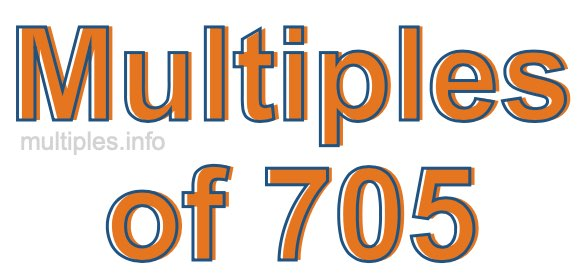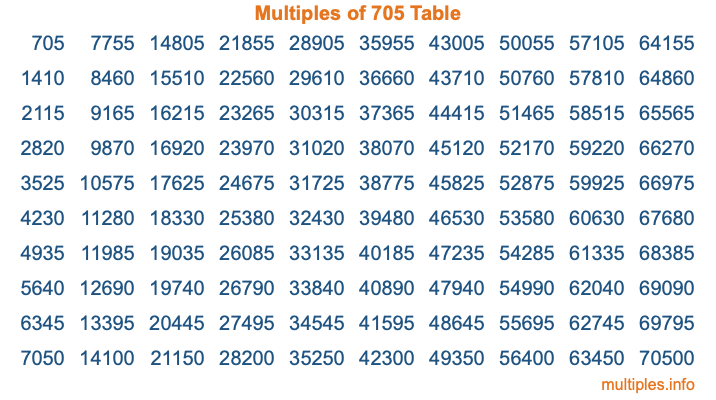Multiples of 705Welcome to the Multiples of 705 page. Here we will first teach you everything you will ever need to know about the multiples of 705, and then give you a study guide summary of everything we taught you to make sure you remember it all. Use this page to look up facts and learn information about the multiples of 705. This page will make you a multiples of seven hundred five expert!

Definition of Multiples of 705
Multiples of 705 are all the numbers that when divided by 705 equal an integer. Each of the multiples of 705 are called a multiple. A multiple of 705 is created by multiplying 705 by an integer.

Therefore, to create a list of multiples of 705, you start with 1 multiplied by 705, then 2 multiplied by 705, then 3 multiplied by 705, and so on for as long as you want. Thus, the list of the first five multiples of 705 is 705, 1410, 2115, 2820, and 3525. To see a larger list of multiples of 705, see the printable image of Multiples of 705 further down on this page. We also have a category where you can choose any nth multiple of 705.

Multiples of 705 Checker
The Multiples of 705 Checker below checks to see if any number of your choice is a multiple of 705. In other words, it checks to see if there is any number (integer) that when multiplied by 705 will equal your number. To do that, we divide your number by 705. If the the quotient is an integer, then your number is a multiple of 705.

Is  a multiple of 705?

Least Common Multiple of 705 and ...
A Least Common Multiple (LCM) is the lowest multiple that two or more numbers have in common. This is also called the smallest common multiple or lowest common multiple and is useful to know when you are adding our subtracting fractions. Enter one or more numbers below (705 is already entered) to find the LCM.

Check out our LCM Calculator if you need more details about the Least Common Multiple or if you need the LCM for different numbers for adding and subtraction fractions.

nth Multiple of 705
As we stated above, 705 is the first multiple of 705, 1410 is the second multiple of 705, 2115 is the third multiple of 705, and so on. Enter a number below to find the nth multiple of 705.

th multiple of 705

Multiples of 705 vs Factors of 705
705 is a multiple of 705 and a factor of 705, but that is where the similarities end. All postive multiples of 705 are 705 or greater than 705. All positive factors of 705 are 705 or less than 705.

Below is the beginning list of multiples of 705 and the factors of 705 so you can compare:

Multiples of 705: 705, 1410, 2115, 2820, 3525, etc.

Factors of 705: 1, 3, 5, 15, 47, 141, 235, 705

As you can see, the multiples of 705 are all the numbers that you can divide by 705 to get a whole number. The factors of 705, on the other hand, are all the whole numbers that you can multiply by another whole number to get 705.

It's also interesting to note that if a number (x) is a factor of 705, then 705 will also be a multiple of that number (x).

Multiples of 705 vs Divisors of 705
The divisors of 705 are all the integers that 705 can be divided by evenly. Below is a list of the divisors of 705.

Divisors of 705: 1, 3, 5, 15, 47, 141, 235, 705

The interesting thing to note here is that if you take any multiple of 705 and divide it by a divisor of 705, you will see that the quotient is an integer.

Multiples of 705 Table
Below is an image of the first 100 multiples of 705 in a table. The table is in chronological order, column by column. The first column has the first ten multiples of 705, the second column has the next ten multiples of 705, and so on.The Multiples of 705 Table is also referred to as the 705 Times Table or Times Table of 705. You are welcome to print out our table for your studies.

Negative Multiples of 705
Although not often discussed or needed in math, it is worth mentioning that you can make a list of negative multiples of 705 by multiplying 705 by -1, then by -2, then by -3, and so on, to get the following list of negative multiples of 705:

-705, -1410, -2115, -2820, -3525, etc.

Multiples of 705 Summary
Below is a summary of important Multiples of 705 facts that we have discussed on this page. To retain the knowledge on this page, we recommend that you read through the summary and explain to yourself or a study partner why they hold true.

There are an infinite number of multiples of 705.

A multiple of 705 divided by 705 will equal a whole number.

705 divided by a factor of 705 equals a divisor of 705.

The nth multiple of 705 is n times 705.

The largest factor of 705 is equal to the first positive multiple of 705.

705 is a multiple of every factor of 705.

705 is a multiple of 705.

A multiple of 705 divided by a divisor of 705 equals an integer.

705 divided by a divisor of 705 equals a factor of 705.

Any integer times 705 will equal a multiple of 705.

Multiples of a Number
Here you can get the multiples of another number, all with the same attention to detail as we did for multiples of 705 on this page.

Multiples of
Multiples of 706
Did you find our page about multiples of seven hundred five educational? Do you want more knowledge? Check out the multiples of the next number on our list!